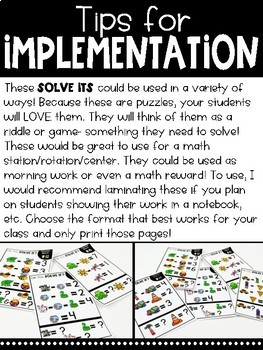# Solve Its: Number Sentence Puzzlers (Vol 2: Subtraction Within 10)Subject
Resource Type
File Type

PDF

(45 MB|67 pages)
Standards
• Product Description
• Standards

This resource is Volume 2 which focuses on subtraction within 10!

What are SOLVE ITS?

Solve Its: Number Sentence Puzzlers are engaging challenges your students will be determined to solve! In each volume, number sentences are provided on each of the 20 Solve Its but instead of numbers being displayed, there are pictures. Students are tasked with using the information provided to solve for the value of each of the pictures.

Each Solve It is provided in a full-page colored version, half-page colored version, half-page black/white version, and printable version with an area for students to show their work. An answer guide is included.

How to USE SOLVE ITS?

Solve Its can be used in many ways in your classroom. You know your students so make the choice that works best for them! These could be used as an independent activity, but would also be a great opportunity for students to collaborate!

→Whole Group Engagement/Warm-up

→Math Center/Rotation/Station

→Morning Work

→Math reward (Introduce these as a reward game/activity for students who do a great job on their work, participate, give a thorough explanation, etc.!)

There are 11 other volumes in the series.

A BUNDLE IS AVAILABLE FOR PURCHASE AT A DEEPLY DISCOUNTED PRICE.

Here is the link to the GROWING BUNDLE.

Volume 1

Volume 2

Volume 3

Volume 4

Volume 5

Volume 6

Volume 7

Volume 8

Volume 9

Volume 10

Volume 11

Volume 12

Fluently add and subtract within 20 using mental strategies. By end of Grade 2, know from memory all sums of two one-digit numbers.
Use addition and subtraction within 100 to solve one- and two-step word problems involving situations of adding to, taking from, putting together, taking apart, and comparing, with unknowns in all positions, e.g., by using drawings and equations with a symbol for the unknown number to represent the problem.
Determine the unknown whole number in an addition or subtraction equation relating three whole numbers. For example, determine the unknown number that makes the equation true in each of the equations 8 + ? = 11, 5 = ▯ - 3, 6 + 6 = ▯.
Solve word problems that call for addition of three whole numbers whose sum is less than or equal to 20, e.g., by using objects, drawings, and equations with a symbol for the unknown number to represent the problem.
Use addition and subtraction within 20 to solve word problems involving situations of adding to, taking from, putting together, taking apart, and comparing, with unknowns in all positions, e.g., by using objects, drawings, and equations with a symbol for the unknown number to represent the problem.
Total Pages
67 pages
Included
Teaching Duration
N/A
Report this Resource to TpT
Reported resources will be reviewed by our team. Report this resource to let us know if this resource violates TpT’s content guidelines.